Courses

# Law of Chemical Equilibrium and Equilibrium Constant Class 11 Notes | EduRev

## Class 11 : Law of Chemical Equilibrium and Equilibrium Constant Class 11 Notes | EduRev

The document Law of Chemical Equilibrium and Equilibrium Constant Class 11 Notes | EduRev is a part of the Class 11 Course Chemistry for JEE.
All you need of Class 11 at this link: Class 11

Equilibrium constant

aA + bB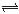cC + dD

At equilibrium, Rf = Rb

kf[A]a [B]b = kr[C]c [D]d →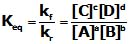Kc → equilibrium constant in terms of concentration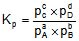(Kp = eq. constant in terms of partial pressure)

for the reaction, N2(g) + 3 H2(g)2NH3(g)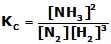,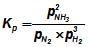Law of Chemical Equilibrium

At a given temperature, the product of concentrations of the reaction products raised to the respective stoichiometric coefficient in the balanced chemical equation divided by the product of concentrations of the reactants raised to their individual stoichiometric coefficients has a constant value. This is known as the equilibrium Law or Law of Chemical Equilibrium

Units of Equilibrium Constant

The value of equilibrium constant KC can be calculated by substituting the concentration terms in mol/L and for Kp partial pressure is substituted in Pa, kPa, bar or atm. This results in units of equilibrium constant based on molarity or pressure. unless the exponents of both the numerator and denominator are same.

For the reactions,

H2(g) + I2(g)2HI, KC and KP have no unit.

N2O4(g)2NO2 (g), KC has unit mol/L and KP has unit bar or atm

Equilibrium constants can also be expressed as dimensionless quantities if the standard state of reactants and products are specified. For a pure gas, the standard state is 1 bar. Therefore a pressure of 4 bar in standard state can be expressed as 4 bar/1 bar = 4, which is a dimensionless number. Standard state (C0) for a solute is 1 molar solution and all concentrations can be measured with respect to it. The numberical value of equilibrium constant depends on the standard state chosen. Thus in this system both Kp and KC are dimensionless quantities and represented as Kp° & KC° respectively

Relation between Kp and Kc

For the reaction aA + bB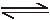cC + dD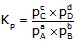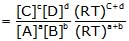Kp=KC(RT)Dng

where Δng = (c +d) - (a +b) = no. of moles of gaseous products - no. of moles of gaseous Reactants

if Δn = 0 Kp = Kc

Significance of Equilibrium Constant:

(a) Using Keq to Predict Relative Concentrations

The size of the equilibrium constant can give us information about the relative amounts of reactants and products present at equilibrium.

• When K << 1
The reaction lies to the left (mostly reactants)

• When K >> 1
The reaction lies to the right (mostly products)

• When K = 1
The reaction lies in the middle (mix of reactants and products)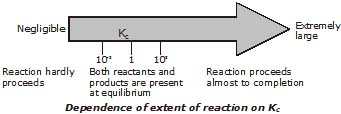(b) Calculating Equilibrium concentrations

Ex. Phosgene is a poisonous gas that dissociates at high temperature into two other poisonous gases, carbon monoxide and chlorine. The equilibrium constant Kp= 0.0041 atm at 600K. Find the equilibrium composition of the system after 0.124 atm of COCl2 initially is allowed to reach equilibrium at this temperature.

Sol.

 COCl2(g) ⇔ CO(g) + Cl2(g) initial pressures 0.124 0 0 change -x +x +x equilibrium pressures 0.124-x x x

Substitution of the equilibrium pressures into the equilibrium expression gives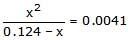This expression can be rearranged into standard polynomial formx2 0041x-0.00054=0 and solved by the quadratic formula, but we will simply obtain an approximate solution by iteration.
Because the equilibrium constant is small, we know that x will be rather small compared to 0.124, so the above relation can be approximated by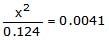which gives x=0.025. To see how good this is, substitute this value of into the denominator of the original equation and solve again: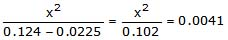This time, solving for x gives 0.0204. Iterating once more, we get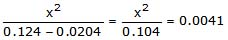and = 0.0206 which is sufficiently close to the previous to be considered the final result. The final partial pressures are then 0.104 atm for COCl2, and 0.0206 atm each for CO and Cl2.

Note: using the quadratic formula to find the exact solution yields the two roots -0.0247 (which we ignore) and 0.0206, which show that our approximation is quite good. Note: using the quadraticformula to find the exact solution yields the two roots -0.0247 (which we ignore) and 0.0206, which show that our approximation is quite good.

Reaction Quotient (Q):

At each point in a reaction, we can write a ratio of concentration terms having the same forms as the equilibrium constant expression. The ratio is called the reaction quotient denoted by symbol Q. It helps in predicting the direction of a reaction.

The expresson Q=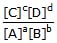at any time during rection is called reaction quotient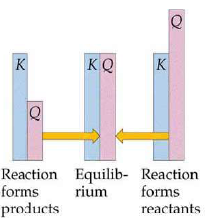(i) if Q > Kreaction will proceed in a bakward direction until equilibrium in reached.

(ii) if Q < Kreaction will proceed in forward direction until equilibrium is established.

(iii) if Q = KC reaction is at equilibrium

Ex. For the reaction NOBr (g)NO(g) + 1/2 Br2(g) , Kp=0.15 atm at 90°C. If NOBr, NO and Br2 are mixed at this temperature having partial pressures 0.5 atm,0.4 atm & 2.0 respectively, will Br2 be consumed or formed?

Sol.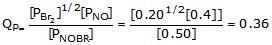KP=0.15

Hence, reaction will shift in backward direction, Therefor Br2 will be consumed

Degree of Dissociation & Vapour Density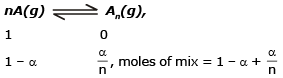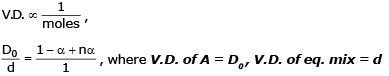Ex. The Vapour Density of mixture of PCl5, PCl3 and Cl2 is 92. Find the degree of dissociation of PCl5.

Sol.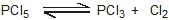1                          0           0
1 - x                      x           x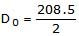= 104.25 ,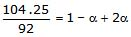,  α =0.13

Characteristics of equilibrium constant

1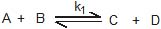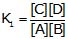After reversing the reaction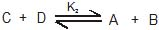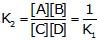After reversing the reaction the equilibrium constant get reversed.

2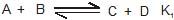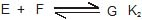and

A + B + E + F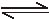C + D + G K3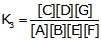→ K3 = K1 × K2

when the two reaction are added there equilibrium constant get multiplied.

3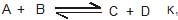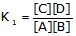After multiplying by n

nA + nBnC + nD K2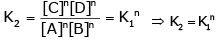When the reaction is multiplied by any number then eq. constant gets the same number in its power.

Ex. Given the following equilibrium constants:

(1) CaCO3(s) → Ca2+ (aq) + CO32-(aq) K1=10-8.4

(2) HCO3-(aq) → H6 (aq) + CO32-(aq) K2=10-10.3

Calculate the value of K for the reaction CaCO3(s) + H (aq)=Ca(aq) + HCO3-(aq)

Sol. The net reaction is the sum of reaction 1 and the reverse of reaction 2:

CaCO3(s) → Ca2  (aq) +CO32-(aq) K1=10-8.4

H+ (aq) + CO32-(aq)→ HCO3-(aq) K-2=10-(-10.3)

-------------------------------------------------

CaCO3(s) + H+ (aq) → Ca(aq) + HCO3-(aq),  K=K1/K2=10(-8.4 +10.3) =10+1.9

Comment: This net reaction describes the dissolution of limestone by acid; it is responsible for the eroding

effect of acid rain on buildings and statues. This is an example of a reaction that has practically no tendency to take place by itself (the dissolution of calcium carbonate) begin "driven" by a second reaction having a large equilibrium constant. From the standpoint of the LeChâtelier principle, the first reaction is "pulled to the right" by the removal of carbonate by the hydrogen ion. "Coupled" reactions of this type are widely encountered in all areas of chemistry, and especially in biochemistry, in which a dozen or so reactions may be linked in this way.

Ex. The synthesis of HBr from hydrogen and liquid bromine has an equilibrium constant Kp = 4.5×1015 at 25°C. Given that the vapor pressure of liquid bromine is 0.28 atm, find Kp for the homogeneous gas-phase reaction at the same temperature.

Sol: The net reaction we seek is the sum of the heterogeneous synthesis and the reverse of the vaporization of liquid bromine: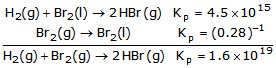Here are some of the possibilities for the reaction involving the equilibrium between gaseous water and its elements: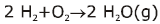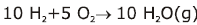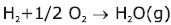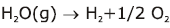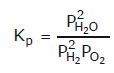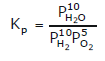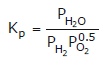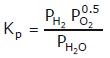Offer running on EduRev: Apply code STAYHOME200 to get INR 200 off on our premium plan EduRev Infinity!

## Chemistry for JEE

223 videos|452 docs|334 tests

,

,

,

,

,

,

,

,

,

,

,

,

,

,

,

,

,

,

,

,

,

;